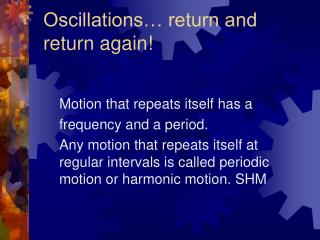DownloadDownload PresentationOscillations… return and return again!

# Oscillations… return and return again!

Download Presentation## Oscillations… return and return again!

- - - - - - - - - - - - - - - - - - - - - - - - - - - E N D - - - - - - - - - - - - - - - - - - - - - - - - - - -
##### Presentation Transcript

1. Oscillations… return and return again! Motion that repeats itself has a frequency and a period. Any motion that repeats itself at regular intervals is called periodic motion or harmonic motion. SHM

2. Hooke’s Law • Vibrational motion of a mass attached to a spring. • Fs = - kx • X = displacement • K is spring constant • - Negative sign means a restoring force

3. Hey Calvin that’s Free fall not periodic motion • The force pulling Calvin down will not put him in a pattern of simple harmonic motion will it?

4. Measuring a spring constant • Fs = kd = mg or • K = mg/d • Simple harmonic motion occurs when the net force along the direction of motion is a Hooke’s law type of force that is, when the net force is proportional to the displacement and in the opposite direction.

5. Define some terms • Amplitude, A – Maximum distance traveled by an object away from its equilibrium position. • Period, T, is the time it takes the object to execute one complete cycle of motion. • Frequency, f, is the number of cycles or vibrations per unit of time.

6. Plotting the position vs time of a particle that oscillates • Exam this at times t = -T/4 through t=T • X(t) = Xmcos(wt + ) • X(t) –displacement at time t. • Xm – Amplitude • w – angular frequency wt +  -- Phase  - phase angle

7. Velocity and Acceleration of SHM • X(t) = Xmcos(wt + ) by differentiation • V(t) = -w xm sin (wt + ) by differentiation • Where w xm = velocity amplitude • A(t) = -w2 xm Cos (wt + ) Where w2 xm = acceleration amplitude • In SHM the acceleration is proportional to the displacement by opposite in sign, and the two quantities are related by the square of the angular frequency.

8. Potential Energy stored in a stretched spring. • F = -kx = ma • A = -k/m *X • PE = ½ kx2 • Energy is stored in a spring only when stretched or when it is compressed. PE is max at max extension.

9. Velocity as function of position • Using conservation of energy we get • 1/2kA2 = ½ mv2 + ½ kx2 • Solving for v we get • v +-  k/m (A2 – x2)

10. Review Position, Velocity, Acceleration as function of time. • W = k/m angular frequeny • T =2 m/k period of mass and spring • T =2 L/g period of simple pendulum

11. Comparing springs and pendulums.

12. Damped SHM • Motion of an oscillator is reduced by an external force • Damping force fd = -bv • B is damping constant. • -bv –kx = ma • Becomes… • X(t) = Xm e-bt/2m Cos (w’t + )

13. What is a wave? • A wave is the motion of a disturbance. • Mechanical waves require a source of disturbance, a medium to propagate along and a physical connection with the influencing medium.

14. Traveling waves….. • Longitudinal- each segment of the rope that is disturbed moves perpendicularly to the wave motion. • Transverse- the particles of the medium undergo displacements parallel to the direction of wave motion

15. The shock heard round the world….. • Read Chapter 13 about waves. • Problems page 434 1-45 odd